Home > INT3 > Chapter 11 > Lesson 11.1.4 > Problem11-42

11-42.
1. Add, subtract, multiply, or divide the following rational expressions. Simplify your answers, if possible. Homework Help ✎

2.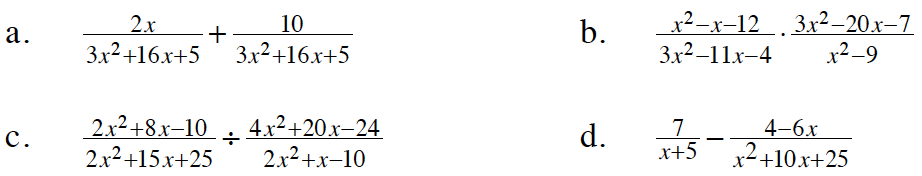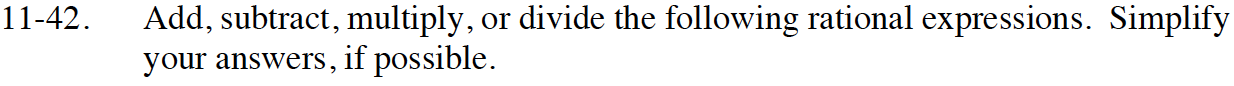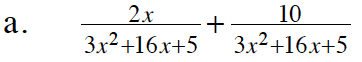These fractions have the same denominator.

After adding the fractions, factor to see if you can find any Giant Ones to remove.

$\frac{2}{3x+1}$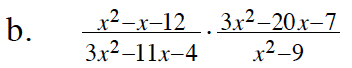Factor each polynomial. Look for Giant Ones to remove.

$\frac{x-7}{x-3}$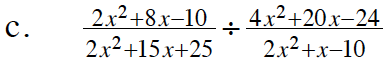Take the reciprocal of the divisor and factor each polynomial. Remove Giant Ones and multiply what's left.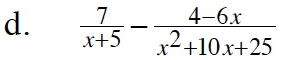Begin by factoring the 2nd denominator and then creating common denominators.

$\frac{x+5}{x + 5} \cdot \frac{7}{x+ 5} - \frac{4 - 6x}{(x+5)(x+5)}$

$\frac{7x+35}{(x+5)(x+5)} - \frac{4-6x}{(x+5)(x+5)}$

$\frac{(7x + 35 )-(4 - 6x)}{(x+5)(x+5)}$

Simplify the numerator.